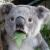《算法之道》精华 经典算法部分

modernizr 发布于 2014/08/11 08:51

《算法之道》精华 经典算法部分

• 本书作者邹恒明，作者另有一本书《数据结构之弦》，以及《操作系统之哲学原理》都是很好的书
• 这本书可以算得上是深入浅出，文笔很好，作者添加了很多自己的思考
• 本文包括经典算法部分

第十章 排序与次序

• 插入排序
• 从无序部分抽取一张插入有序部分
• 为原地排序，无需占用临时存储空间
• 最优情况下为O(n)，平均O(n^2)
• 折半插入排序
• 插入时使用二分查找
• 归并排序
• 分治，从中间分解，分别排序后进行仔细的合并
• 异地排序，需要占用额外空间
• n>=30时性能比插入排序更好。复杂度固定为O(nlog(n))
• 快排
• 分治，复杂的部分在于分解，而归并复杂在于合并
• 原地排序
• 最坏情况为O(n^2)，但只要不是每次都是最坏，复杂度就不是n^2，具有韧性
• 任何基于比较的排序，决策树高度至少为nlog(n)
• 计数排序
• 元素值范围必须有限
• 空间复杂度高
• O(n)
• 基数排序
• 从最低位到最高位排序，每一位排序都采用稳定排序，如计数排序
• 一位排序应该选择log(n)个比特，使整体成本最低
• 桶排序
• 把n元素按值分到n个桶里，每个桶内部进行插入排序，将各桶首位相连
• 元素应该是均匀分布
• 快速次序选择：求第K大的数
• 使用快排的partition
• 最差O(n^2)，平均O(n)
• 线性最差快速次序选择
• 将元素每5个一组，分别取中值。在n/5个中值里面找到中值，作为partition的pivot
• 为什么*不每3个一组？
• 保证pivot左边右边至少3n/10个元素
• 最差O(n)

第十一章 搜索与散列

• 顺序搜索
• 在序列里面如果搜索频率从头到尾指数递减，则为O(1)
• 折半搜索
• 对于有序序列，为O(logn)
• 常数搜索：散列搜索
• 直接散列：非常简单，不会发生碰撞，空间浪费大
• 除法（模除法）散列
• 元素对散列表大小m取模得到
• m必须为素数，否则造成不均匀散射。比如m包含因子d，而大部分元素对d余数相等
• m不能靠近2的幂。如m为2的幂，散列结果将不依赖元素的所有位。靠近也不行，为什么
• 乘法散列
• h(k) = (A * k ) % 2^r >> (w - r)，w为计算机字宽，A为2^(w-1)与2^w之间的一个奇数
• 乘方取中法：乘方n次（常取n=2），取中间r位
• 开放寻址散列：散列碰撞时纵深扩展，添加一个链表
• 平均搜索时间为O(1+a)，a为加载因子
• 封闭寻址散列：散列碰撞时为元素找到另一个位置
• 找另一个位置的操作称为探寻
• 线性探寻
• h(k,i) = (h'(k) + i) % m，h'(k)为家位
• 向单方向寻找未被占用的位置
• 易出现顶级聚集
• 非线性探寻
• 平方探寻 h(k,i) = (h'(k) + c1 * i + c2 * i^2) % m 易出现次级聚集
• 双重散列探寻
• 使用两个散列函数h1、h2来构造新散列函数
• h(k,i) = (h1(k) + i * h2(k) ) mod m
• 伪随机探寻
• 使用伪随机序列
• 存在次级聚集
• 不成功搜索的探寻次数期望为1/(1-a)
• 成功搜索探寻次数最多为1 / a * ln( 1/(1-a))
• 封闭散列不能删除元素，可以放标记解决。如果插入相比搜索非常稀疏，则可以通过重新散列解决空位问题
• 随机化散列
• 找到一组散列函数，每次随机选择一个不同的散列函数
• 用于避免单个散列函数极端情况下聚集效应严重
• 全域散列
• 一组H个散列函数，将任意两个不同的元素映射到同一位置的函数个数为H/m
• 完美散列
• n个元素，构造m=O(n)大小的散列表，使搜索最坏达到O(1)
• 采用双层散列，第一层大小n，第二层每个表的大小为落到第一层位置i上的元素个数的平方
• 空间消耗为O(n)

第十二章 最短路径

• 如果图中有负环，则不存在最短路径
• 单源多点最短路径
• Dijkstra算法
• 贪婪算法，要求不存在负路径
• 最优子结构：最短路径里的每一段都是两点之间的最短路径
• 贪婪选择属性：路径向外延伸的下一个节点就是离源点最近的节点
• 每次选取离源点最近的节点，更新所有与此节点相邻节点的距离
• 时间复杂度为O(V^2)，采用堆实现，可以达到O(E log(V))。与Prim算法相同
• Bellman-Ford算法
• 可以应对负权重
• 进行V-1轮降距，每次更新图中所有边
• 复杂度为O(VE)
• BFS
• 各边权重相等的情况
• O(V+E)
• 多源多点最短路径
• Floyd-Warshall算法
• 动态规划算法
• 子问题为从i到j，中间结点只属于集合1...k的最短路径长度
• c_ijk = min{c_ij(k-1), c_ik(k-1) + ckj(k-1)}|k
• 复杂度O(n^3)
• Jonhson算法
• 等效变换为无负权重的图，使用Dijkstra算法
• 添加一个节点s，到所有点路径长度为0，运行Bellman-Ford算法，对节点赋值
• 对每个节点运行Dijkstra算法
• 复杂度主要是Dijkstra算法运算，为O(VE + V^2 log(V))
• 若Bellman-Ford算法报告有负环存在，不能使用此方法

0